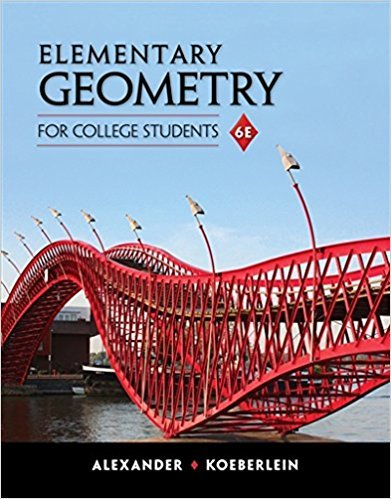# Solutions for Chapter 7: Locus and Concurrence## Full solutions for Elementary Geometry for College Students | 6th Edition

ISBN: 9781285195698Solutions for Chapter 7: Locus and Concurrence

Solutions for Chapter 7
4 5 0 271 Reviews
29
5
##### ISBN: 9781285195698

Elementary Geometry for College Students was written by and is associated to the ISBN: 9781285195698. Chapter 7: Locus and Concurrence includes 46 full step-by-step solutions. Since 46 problems in chapter 7: Locus and Concurrence have been answered, more than 2418 students have viewed full step-by-step solutions from this chapter. This textbook survival guide was created for the textbook: Elementary Geometry for College Students, edition: 6. This expansive textbook survival guide covers the following chapters and their solutions.

Key Math Terms and definitions covered in this textbook
• Affine transformation

Tv = Av + Vo = linear transformation plus shift.

• Associative Law (AB)C = A(BC).

Parentheses can be removed to leave ABC.

• Big formula for n by n determinants.

Det(A) is a sum of n! terms. For each term: Multiply one entry from each row and column of A: rows in order 1, ... , nand column order given by a permutation P. Each of the n! P 's has a + or - sign.

• Characteristic equation det(A - AI) = O.

The n roots are the eigenvalues of A.

• Column space C (A) =

space of all combinations of the columns of A.

• Elimination matrix = Elementary matrix Eij.

The identity matrix with an extra -eij in the i, j entry (i #- j). Then Eij A subtracts eij times row j of A from row i.

• Four Fundamental Subspaces C (A), N (A), C (AT), N (AT).

Use AT for complex A.

• Full column rank r = n.

Independent columns, N(A) = {O}, no free variables.

• Graph G.

Set of n nodes connected pairwise by m edges. A complete graph has all n(n - 1)/2 edges between nodes. A tree has only n - 1 edges and no closed loops.

• Hankel matrix H.

Constant along each antidiagonal; hij depends on i + j.

• Indefinite matrix.

A symmetric matrix with eigenvalues of both signs (+ and - ).

• Linearly dependent VI, ... , Vn.

A combination other than all Ci = 0 gives L Ci Vi = O.

• Orthonormal vectors q 1 , ... , q n·

Dot products are q T q j = 0 if i =1= j and q T q i = 1. The matrix Q with these orthonormal columns has Q T Q = I. If m = n then Q T = Q -1 and q 1 ' ... , q n is an orthonormal basis for Rn : every v = L (v T q j )q j •

• Projection matrix P onto subspace S.

Projection p = P b is the closest point to b in S, error e = b - Pb is perpendicularto S. p 2 = P = pT, eigenvalues are 1 or 0, eigenvectors are in S or S...L. If columns of A = basis for S then P = A (AT A) -1 AT.

• Reduced row echelon form R = rref(A).

Pivots = 1; zeros above and below pivots; the r nonzero rows of R give a basis for the row space of A.

• Right inverse A+.

If A has full row rank m, then A+ = AT(AAT)-l has AA+ = 1m.

• Singular Value Decomposition

(SVD) A = U:E VT = (orthogonal) ( diag)( orthogonal) First r columns of U and V are orthonormal bases of C (A) and C (AT), AVi = O'iUi with singular value O'i > O. Last columns are orthonormal bases of nullspaces.

• Symmetric factorizations A = LDLT and A = QAQT.

Signs in A = signs in D.

• Vector v in Rn.

Sequence of n real numbers v = (VI, ... , Vn) = point in Rn.

• Volume of box.

The rows (or the columns) of A generate a box with volume I det(A) I.

×

I don't want to reset my password

Need help? Contact support

Need an Account? Is not associated with an account
We're here to help